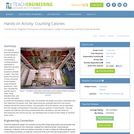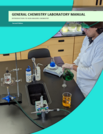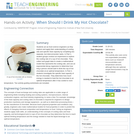Updating search results...

# 3 Results

View
Selected filters:
• calorimeterEducational Use
Rating
0.0 stars

The students discover the basics of heat transfer in this activity by constructing a constant pressure calorimeter to determine the heat of solution of potassium chloride in water. They first predict the amount of heat consumed by the reaction using analytical techniques. Then they calculate the specific heat of water using tabulated data, and use this information to predict the temperature change. Next, the students will design and build a calorimeter and then determine its specific heat. After determining the predicted heat lost to the device, students will test the heat of solution. The heat given off by the reaction can be calculated from the change in temperature of the water using an equation of heat transfer. They will compare this with the value they predicted with their calculations, and then finish by discussing the error and its sources, and identifying how to improve their design to minimize these errors.

Subject:
Applied Science
Engineering
Physical Science
Physics
Material Type:
Activity/Lab
Provider:
TeachEngineering
Provider Set:
TeachEngineering
Author:
James Prager
Janet Yowell
Malinda Zarske
Megan Schroeder
09/18/2014Conditional Remix & Share Permitted
CC BY-NC
Rating
0.0 stars

This laboratory manual is an introduction to a standard chemical lab course for Arizona Community Colleges CHEM 130. It covers basic protocols, glassware, and handling techniques. Students will be presented with full immersive experiences to enrich their understanding of basic chemistry.

Subject:
Chemistry
Material Type:
Activity/Lab
Author:
Eric Osborn
05/03/2022Educational Use
Rating
0.0 stars

Students act as food science engineers as they explore and apply their understanding of cooling rate and specific heat capacity by completing two separate, but interconnected, tasks. In Part 1, student groups conduct an experiment to explore the cooling rate of a cup of hot chocolate. They collect and graph data to create a mathematical model that represents the cooling rate, and use an exponential decay regression to determine how long a person should wait to drink the cup of hot chocolate at an optimal temperature. In Part 2, students investigate the specific heat capacity of the hot chocolate. They determine how much energy is needed to heat the hot chocolate to an optimal temperature after it has cooled to room temperature. Two activity-guiding worksheets are included.

Subject:
Algebra
Chemistry
Mathematics
Physical Science
Statistics and Probability
Material Type:
Activity/Lab
Provider:
TeachEngineering
Provider Set:
TeachEngineering
Author:
Brian Palacios